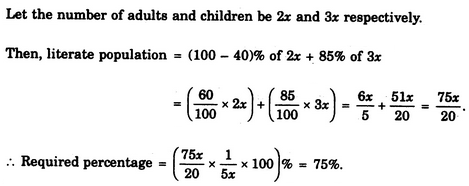# Verbal Reasoning - Arithmetic Reasoning

### Exercise :: Arithmetic Reasoning - Section 1

26.

Five bells begin to toll together and toll respectively at intervals of 6, 5, 7, 10 and 12 seconds. How many times will they toll together in one hour excluding the one at the start ?

 A. 7 times B. 8 times C. 9 times D. 11 times

Explanation:

L.C.M. of 6, 5, 7, 10 and 12 is 420.

So, the bells will toll together after every 420 seconds i.e. 7 minutes.

Now, 7 x 8 = 56 and 7 x 9 = 63.

Thus, in 1-hour (or 60 minutes), the bells will toll together 8 times, excluding the one at the start.

27.

A bus starts from city X. The number of women in the bus is half of the number of men. In city Y, 10 men leave the bus and five women enter. Now, number of men and women is equal. In the beginning, how many passengers entered the bus ?

 A. 15 B. 30 C. 36 D. 45

Explanation:

Originally, let number of women = x. Then, number of men = 2x.

So, in city Y, we have : (2x - 10) = (x + 5) or x - 15.

Therefore Total number of passengers in the beginning = (x + 2x) = 3x = 45.

28.

A, B, C, D and E play a game of cards. A says to B, "If you give me 3 cards, you will have as many as I have at this moment while if D takes 5 cards from you, he will have as many as E has." A and C together have twice as many cards as E has. B and D together also have the same number of cards as A and C taken together. If together they have 150 cards, how many cards has C got ?

 A. 28 B. 29 C. 31 D. 35

Explanation:

Clearly, we have :

A = B - 3 ...(i)

D + 5 = E ...(ii)

A+C = 2E ...(iii)

B + D = A+C = 2E ...(iv)

A+B + C + D + E=150 ...(v)

From (iii), (iv) and (v), we get: 5E = 150 or E = 30.

Putting E = 30 in (ii), we get: D = 25.

Putting E = 30 and D = 25 in (iv), we get: B = 35.

Putting B = 35 in (i), we get: A = 32.

Putting A = 32 and E = 30 in (iii), we get: C = 28.

29.

A farmer built a fence around his square plot. He used 27 fence poles on each side of the square. How many poles did he need altogether ?

 A. 100 B. 104 C. 108 D. None of these

Explanation:

Since each pole at the corner of the plot is common to its two sides, so we have :

Total number of poles needed = 27 x 4 - 4 = 108 - 4 = 104.

30.

In a city, 40% of the adults are illiterate while 85% of the children are literate. If the ratio of the adults to that of the children is 2 : 3, then what percent of the population is literate ?

 A. 20% B. 25% C. 50% D. 75%